Normally, once an expression has been created in a Maple session, it is always written in the same order. Thus if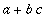has occurred previously, even as a sub-expression (e.g. in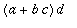), and you enter c*b+a , Maple will rewrite it as.

You can use
sort to change the order of an expression. The first argument to sort is the expression to sort, and the second is a list of variables or functions. A third argument plex causes the sorting to be in pure lexicographic order in the members of the list, rather than decreasing total degree in the members of the list.

After being sorted, the expression stays in the sorted order.

> sort(a+b*c+c^3*d, [b,c]);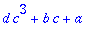This is in total-degree order, so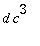, which is of order 3 in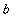and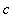, comes before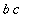which is of order 2 in those variables.

> sort(a+b*c+c^3*d, [b,c],plex);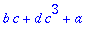In pure lexicographic order in [b,c] ,comes beforebecause b has first priority. In a sum, terms containing no members of the list come last. In a product, however, factors containing no members of the list come first. Thus we obtainedrather than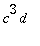.

However, suppose you have the expression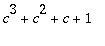and wish to change it to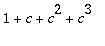. There is no way to use sort to do this. You would have to create the expression initially as 1 + c + c^2 + c^3 . But there is a trick you can use: obtain a new variable that looks like c but is not the same for Maple, and create the expression using that new variable. Then assign the new variable as a value to c .

> p:= c^3 + c^2 + c + 1;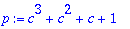> 1 + newc + newc^2 + newc^3;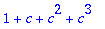> c:= newc;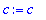> p;In a more complicated case, to create the new version of the expression you could first convert the expression to a list, permute the elements as desired, substitute newc for c , and then convert back to a sum.

> plist:= convert(p,list);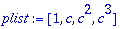> [op(plist[2..4]), plist];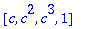> subs(c=newc,");> convert(",`+`);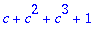> c:= newc;> p;Maple Advisor Database R. Israel, 1997